Preface¶

This is a graduate-level textbook about algorithms for computing with modular forms. It is nontraditional in that the primary focus is not on underlying theory; instead, it answers the question how do you use a computer to explicitly compute spaces of modular forms?

This book emerged from notes for a course the author taught at Harvard University in 2004, a course at UC San Diego in 2005, and a course at the University of Washington in 2006.

The author has spent years trying to find good practical ways to compute with classical modular forms for congruence subgroups of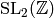and has implemented most of these algorithms several times, first in C++ [Ste99b], then in MAGMA [BCP97], and as part of the free open source computer algebra system Sage (see [Ste06]). Much of this work has involved turning formulas and constructions buried in obscure research papers into precise computational recipes then testing these and eliminating inaccuracies.

The author is aware of no other textbooks on computing with modular forms, the closest work being Cremona’s book [Cre97a], which is about computing with elliptic curves, and Cohen’s book [Coh93] about algebraic number theory.

In this book we focus on how to compute in practice the spaces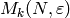of modular forms, where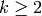is an integer and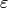is a Dirichlet character of modulus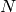(the appendix treats modular forms for higher rank groups). We spend the most effort explaining the general algorithms that appear so far to be the best (in practice!) for such computations. We will not discuss in any detail computing with quaternion algebras, half-integral weight forms, weight 1 forms, forms for noncongruence subgroups or groups other than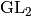, Hilbert and Siegel modular forms, trace formulas,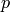-adic modular forms, and modular abelian varieties, all of which are topics for additional books. We also rarely analyze the complexity of the algorithms, but instead settle for occasional remarks about their practical efficiency.

For most of this book we assume the reader has some prior exposure to modular forms (e.g., [DS05]), though we recall many of the basic definitions. We cite standard books for proofs of the fundamental results about modular forms that we will use. The reader should also be familiar with basic algebraic number theory, linear algebra, complex analysis (at the level of [Ahl78]), and algorithms (e.g., know what an algorithm is and what big oh notation means). In some of the examples and applications we assume that the reader knows about elliptic curves at the level of [Sil82].

Chapter Modular Forms is foundational for the rest of this book. It introduces congruence subgroups ofand modular forms as functions on the complex upper half plane. We discuss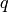-expansions, which provide an important computational handle on modular forms. We also study an algorithm for computing with congruence subgroups. The chapter ends with a list of applications of modular forms throughout mathematics.

In Chapter Modular Forms of Level 1 we discuss level 1 modular forms in much more detail. In particular, we introduce Eisenstein series and the cusp form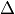and describe their-expansions and basic properties. Then we prove a structure theorem for level 1 modular forms and use it to deduce dimension formulas and give an algorithm for explicitly computing a basis. We next introduce Hecke operators on level 1 modular forms, prove several results about them, and deduce multiplicativity of the Ramanujan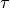function as an application. We also discuss explicit computation of Hecke operators. In Section Fast Computation of Fourier Coefficients we make some brief remarks on recent work on asymptotically fast computation of values of. Finally, we describe computation of constant terms of Eisenstein series using an analytic algorithm. We generalize many of the constructions in this chapter to higher level in subsequent chapters.

In Chapter Modular Forms of Weight 2 we turn to modular forms of higher level but restrict for simplicity to weight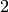since much is clearer in this case. (We remove the weight restriction later in Chapter General Modular Symbols.) We describe a geometric way of viewing cuspidal modular forms as differentials on modular curves, which leads to modular symbols, which are an explicit way to present a certain homology group. This chapter closes with methods for explicitly computing cusp forms of weight 2 using modular symbols, which we generalize in Chapter Computing with Newforms.

In Chapter Dirichlet Characters we introduce Dirichlet characters, which are important both in explicit construction of Eisenstein series (in Chapter Eisenstein Series and Bernoulli Numbers) and in decomposing spaces of modular forms as direct sums of simpler spaces. The main focus of this chapter is a detailed study of how to explicitly represent and compute with Dirichlet characters.

Chapter Eisenstein Series and Bernoulli Numbers is about how to explicitly construct the Eisenstein subspace of modular forms. First we define generalized Bernoulli numbers attached to a Dirichlet character and an integer then explain a new analytic algorithm for computing them (which generalizes the algorithm in Chapter Modular Forms of Level 1). Finally we give without proof an explicit description of a basis of Eisenstein series, explain how to compute it, and give some examples.

Chapter Dimension Formulas records a wide range of dimension formulas for spaces of modular forms, along with a few remarks about where they come from and how to compute them.

Chapter Linear Algebra is about linear algebra over exact fields, mainly the rational numbers. This chapter can be read independently of the others and does not require any background in modular forms. Nonetheless, this chapter occupies a central position in this book, because the algorithms in this chapter are of crucial importance to any actual implementation of algorithms for computing with modular forms.

Chapter General Modular Symbols is the most important chapter in this book; it generalizes Chapter Modular Forms of Weight 2 to higher weight and general level. The modular symbols formulation described here is central to general algorithms for computing with modular forms.

Chapter Computing with Newforms applies the algorithms from Chapter General Modular Symbols to the problem of computing with modular forms. First we discuss decomposing spaces of modular forms using Dirichlet characters, and then explain how to compute a basis of Hecke eigenforms for each subspace using several approaches. We also discuss congruences between modular forms and bounds needed to provably generate the Hecke algebra.

Chapter Computing Periods is about computing analytic invariants of modular forms. It discusses tricks for speeding convergence of certain infinite series and sketches how to compute every elliptic curve over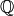with given conductor.

Chapter Solutions to Selected Exercises contains detailed solutions to most of the exercises in this book. (Many of these were written by students in a course taught at the University of Washington.)

Appendix Computing in Higher Rank deals with computational techniques for working with generalizations of modular forms to more general groups than, such as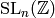for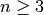. Some of this material requires more prerequisites than the rest of the book. Nonetheless, seeing a natural generalization of the material in the rest of this book helps to clarify the key ideas. The topics in the appendix are directly related to the main themes of this book: modular symbols, Manin symbols, cohomology of subgroups ofwith various coefficients, explicit computation of modular forms, etc.

Software¶

We use Sage, Software for Algebra and Geometry Experimentation (see [Ste06]), to illustrate how to do many of the examples. Sage is completely free and packages together a wide range of open source mathematics software for doing much more than just computing with modular forms. Sage can be downloaded and run on your computer or can be used via a web browser over the Internet. The reader is encouraged to experiment with many of the objects in this book using Sage. We do not describe the basics of using Sage in this book; the reader should read the Sage tutorial (and other documentation) available at the Sage website [Ste06]. All examples in this book have been automatically tested and should work exactly as indicated in Sage version at least 1.5.

Acknowledgements¶

David Joyner and Gabor Wiese carefully read the book and provided a huge number of helpful comments.

John Cremona and Kevin Buzzard both made many helpful remarks that were important in the development of the algorithms in this book. Much of the mathematics (and some of the writing) in Chapter Computing Periods is joint work with Helena Verrill.

Noam Elkies made remarks about Chapters Modular Forms and Modular Forms of Level 1. Sándor Kovács provided interesting comments on Chapter Modular Forms. Allan Steel provided helpful feedback on Chapter Linear Algebra. Jordi Quer made useful remarks about Chapter Dirichlet Characters and Chapter Dimension Formulas.

H. Stark suggested using Stirling’s formula in Section The Number of Digits of , and Mark Watkins and Lynn Walling made comments on Chapter Modular Forms of Weight 2.

Justin Walker found typos in the first published version of the book.

Parts of Chapter Modular Forms follow Serre’s beautiful introduction to modular forms [Ser73, Ch. VII] closely, though we adjust the notation, definitions, and order of presentation to be consistent with the rest of this book.

I would like to acknowledge the partial support of NSF Grant DMS 05-55776. Gunnells was supported in part by NSF Grants DMS 02-45580 and DMS 04-01525.

Notation and Conventions¶

We denote canonical isomorphisms by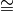and noncanonical isomorphisms by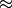. If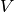is a vector space and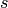denotes some sort of construction involving, we let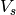denote the corresponding subspace and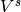the quotient space. E.g., ifis an involution of, then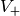is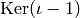and. If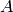is a finite abelian group, then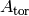denotes the torsion subgroup and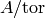denotes the quotient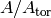. We denote right group actions using exponential notation. Everywhere in this book,is a positive integer and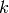is an integer.

Ifis an integer, a divisorofis a positive integer such that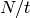is an integer.

Previous topic

Modular Forms, A Computational Approach

Modular Forms